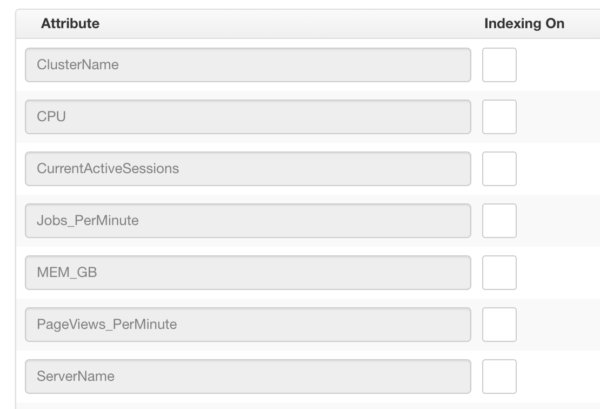Indexing

## Overview

edgeCore 3.2 introduced the concept of indexing, which can drastically enhance the performance of some queries. The concept is exposed as check boxes next to the attribute names in the resulting dataset.To enable indexing, simply click the check box next to the name of the attribute. Each checked attribute will have an index created for that specific attribute.

Any query using this dataset as a source can only use one index. Adding indexes on other attributes will not help.

## Best Practices

Indexing will boost performance in certain situations, but it can hurt performance if an index is created but cannot be used in a transform that references the dataset with the index. Use an index if:

• The dataset the index is added to is referenced by a SQL Transform
• That SQL Transform has a clause such as the following:
(example assumes the ServerName attribute from the image above was indexed):WHERE ServerName = {nodeVar.someVar}
• There is only a single comparison in the WHERE clause. See below for how to deal with multiple comparisons.

If the dataset with the indexing is referenced by multiple SQL Transforms, each filtering on a different attribute in the WHERE clause, adding multiple indexes would then be useful. Each index will only help if the conditions above match, however.

### Dealing with multiple comparisons

If multiple comparisons are needed in a transform, an index will only be used if the SQL can be organized to have a nested select where the interior select meets the requirements above. The following example demonstrates this, where AlertGroup has an index in the dataset ‘incidents’:

```select * from (